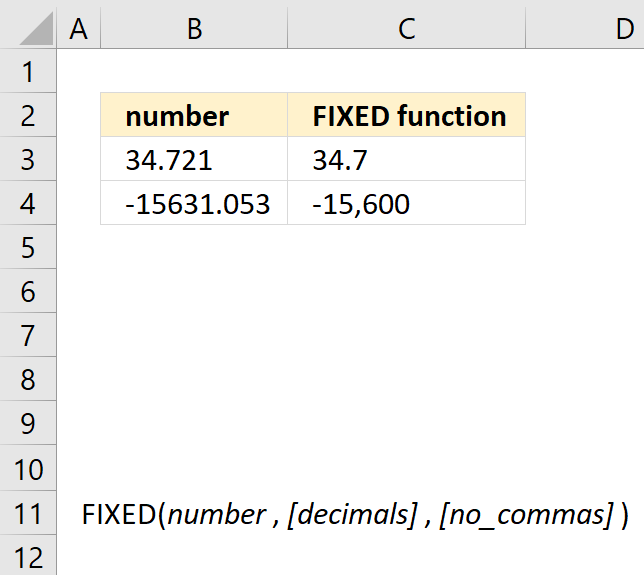Author: Oscar Cronquist Article last updated on May 04, 2022The FIXED function rounds a number to the specified number of decimals, formats the number in decimal format using a period and commas, and returns the result as text.

Formula in cell C3:

=FIXED(B3,1)

Formula in cell C4:

=FIXED(B4,-2)

### Excel Function Syntax

FIXED(number, [decimals], [no_commas])

### Arguments

 number Required. The number you want to round and convert to text. [decimals] Optional. The number of digits to the right of the decimal point. Negative numbers to the left of the decimal point. [no_commas] Optional.  Default value is FALSE. TRUE returns no commas in text string.